# What is the Break Even Formula?The break even formula is used to calculate the point at which revenues equal costs, known as the break even point. It is the point at which a business “breaks even,” or recovers the amount of money that has been put into the business.

Some resources may also refer to it as the "breakeven point" or hyphenate the two words but this difference is trivial.

The break even point is the point at which a business may begin to turn a profit. Up until this point, costs have outpaced revenues.

## Calculating The Break Even Point

Profit is surplus revenue. There cannot be any profit to collect while costs continue to exceed revenues.

The break even point represents the point at which revenues equal costs.

There is no surplus revenue, so there is also no profit, but sales after the break even point will generate profit. Keep in mind, the break even point is by no means a guaranteed milestone for any business.

To accurately calculate the break even point of a venture (or a product line, or a business unit, etc.) you must have a complete picture of both fixed and variable costs. Together, these costs paint a picture of the total expenses for a business.

With this information we can conduct a break even analysis and understand how feasible it is that a business, product, or project will break even and hopefully go on to turn a profit.

### Fixed Costs

Fixed costs are costs that do not change with the number of units produced or sold—things like rent, salaries, or insurance. For the breakeven calculation, use a total of all fixed costs.

### Variable Costs

Variable costs are costs that do change with the number of units produced or units sold.

Service-based businesses that don't sell physical products record variable costs as costs that change with the number of services executed.

These costs include production materials, components, supplies, and other consumables directly tied to production or service execution.

For the break even point calculation, variable costs are the total costs on a per-unit basis.

To determine the point in units, use the following break even point formula:Note:

• Fixed costs are the total fixed costs for the period.
• The price is the per-unit selling price
• Variable costs are the total per-unit cost

The denominator of the breakeven equation is known as the contribution margin. The contribution margin is the amount of money available from each sale that is left over to pay the company’s fixed costs. Once the break even point has been met, the contribution margin also tells us how much of each sale contributes to total profit.

To find the break even point in units, divide fixed costs by the contribution margin.

Let’s take a look at an example of the break even formula in action.

## The Break Even Equation in Action

An online retailer adds up her fixed costs including hosting for her online store and rent and utilities on the space she uses to store her inventory.

Total fixed costs for an average month come to \$3,980.

Variable costs, including the cost to purchase and ship the products she sells, total \$7.46 per unit, and she wants to sell them for \$13.99 each.

With our values plugged into the formula, the break even calculation appears as follows: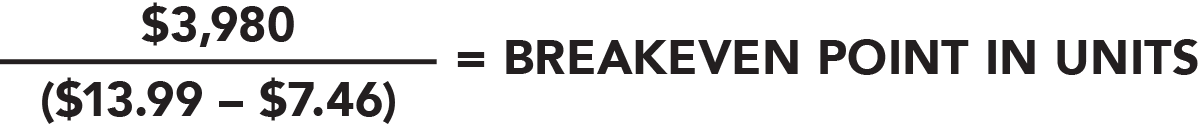Following the order of operations and solving the contribution margin in the equation’s denominator, we are left with the following formula that is easily solved.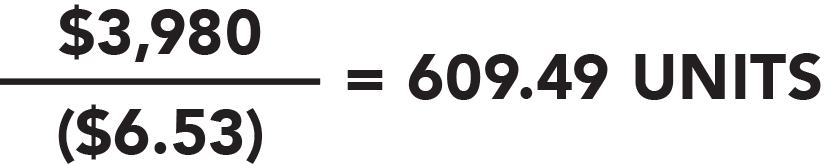Because our fixed costs were totaled for the period of a single month, we can see that our online retailer would have to sell 610 units (no one is interested in purchasing half a unit) in a month to break even.

If up until now sales averaged four hundred units per month, for example, that would be a worrisome sign.

By substituting different numbers for each of the pieces of the equation—fixed costs, variable costs, and sales price—it is possible to model the effects of different strategic options.

What would happen if you were able to cut your variable costs by even just nine cents per unit?

How much lower would the sales threshold be before you were able to break even in a month, a quarter, or a year?

If you are considering lowering your sales price, how many more units would you have to sell in a period to cover your fixed costs?

Simply swap out the numbers in the equation and you will have your answer to any of the above questions.

## Calculating The Break Even Point In Dollars

To calculate the break even point in dollars instead of units we first need to know the contribution ratio. If we already know our contribution margin, and we do from the example above, it is easy to calculate the contribution margin ratio.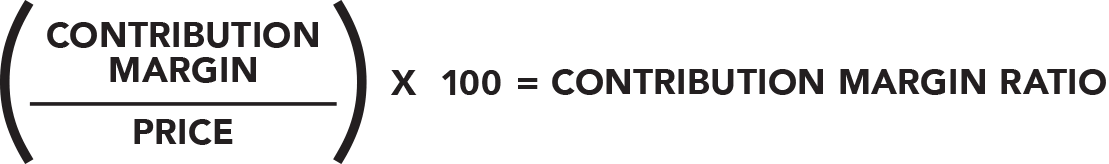Remember, the contribution margin is the denominator of the breakeven formula, or the price per unit minus the total variable costs per unit.

Once the contribution margin ratio has been calculated, it is plugged into the formula below to determine the break even point in sales dollars.Thinking back to our online retailer example, let’s calculate the same break even point in dollars instead of units.

In determining the number of units needed to break even for the month, we calculated the contribution margin to be \$6.53 and we know that the sales price per unit is \$13.99.

Plugging those numbers into our contribution margin ratio, we get a result of 46.68 percent.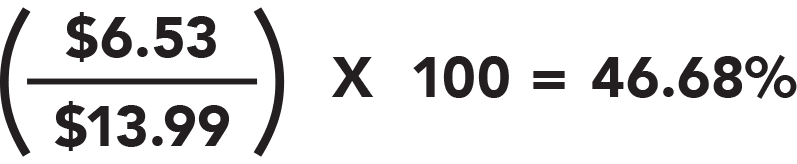The next step is to use our formula for the breakeven point in dollars. This requires the number we just calculated as well as the total fixed costs for the period. Looking back at the example, we know total fixed costs come to \$3,980 for the month.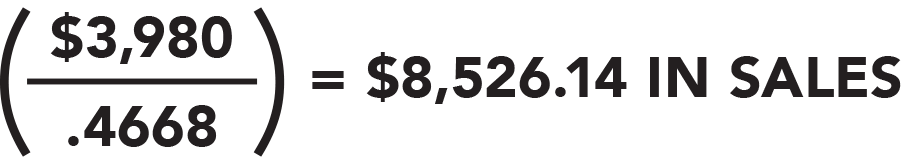As we can see, the break even point will be reached when the retailer makes about \$8,530 in sales.

Keep in mind, however, that just meeting the break even point will not produce the profit necessary to sustain the business. At the break even point revenues equal costs meaning there isn't any profit until this point has been surpassed.

### How Many Units Do I Have To Sell To Achieve \$X In Profit?

A break even analysis can also be used to determine the feasibility of hitting specific profit thresholds. By swapping some of the numbers in the formula, we can see how many units we would need to sell to achieve a desired profit.

If our example online retailer wants to determine the number of sales needed to reach a profit of \$1,200 per month, she only needs to add the desired profit to the fixed costs in the equation.

By treating the desired profit threshold as a “fixed cost,” the same formula can be applied using the combined total of \$5,180 (known fixed costs of \$3,980 plus the desired profit of \$1,200) to determine how many sales will achieve the desired profit.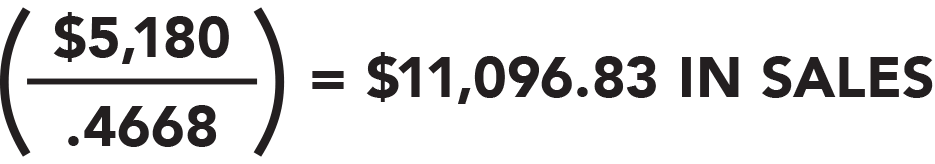## Featured Book

• Email
• More Networks
[class^="wpforms-"]
[class^="wpforms-"]
[class^="wpforms-"]
[class^="wpforms-"]
[class^="wpforms-"]
[class^="wpforms-"]
[class^="wpforms-"]
[class^="wpforms-"]
[class^="wpforms-"]
[class^="wpforms-"]
[class^="wpforms-"]
[class^="wpforms-"]
[class^="wpforms-"]
[class^="wpforms-"]
[class^="wpforms-"]
[class^="wpforms-"]
[class^="wpforms-"]
[class^="wpforms-"]
[class^="wpforms-"]
[class^="wpforms-"]
[523.251,1046.50]
[523.251,1046.50]
[523.251,1046.50]
[523.251,1046.50]
[523.251,1046.50]
[523.251,1046.50]
Share via